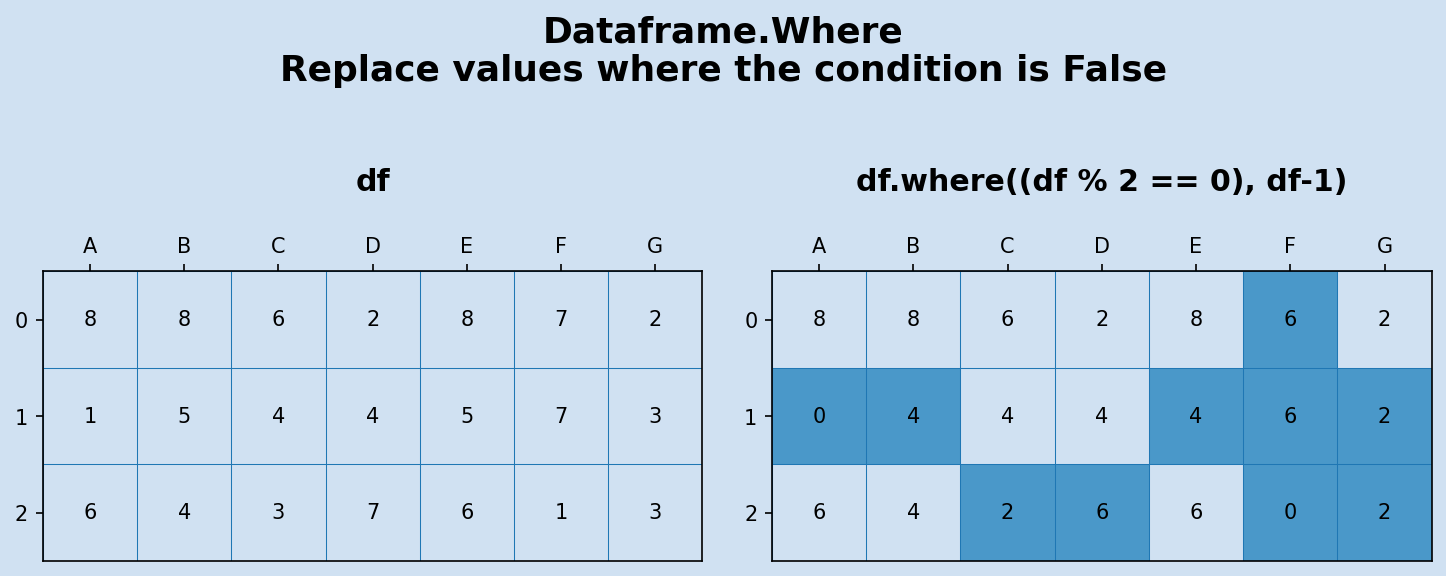# Pandas - Dataframe Where function

The Where method of Pandas Dataframe is used to replace data in a dataframe where the condition is false. It can return a new dataframe or modify the data in the original dataframe, thereby saving memory and object creation.

The syntax of the Dataframe Where function can be confusing. At a minumum it requires just one parameter - the condition to apply to the dataframe, but it replaces all the data where the condition is false. It can also be used with two parameters where the first parameter is the condition and the second is a value or an operation to be applied when the condition is false.

DataFrame.where(cond, other=nan, inplace=False, axis=None, level=None, errors='raise', try_cast=False)

## Where

Pandas dataframe where function is used to replace data in a dataframe where the condition is false. It is not analogous to the Where clause in SQL databases, which is used to filter on data where the condition is true. It is easier to demonstrate with a couple of examples.

## Where numbers are even

Create a condition to identify even numbers in the dataframe. The following code applies modulo 2 to each element in the dataframe and shows true where the result is equal to 0.

Create a dataframe.

 1# Create Dataframe
2np.random.seed(2)
3df = pd.DataFrame(np.random.randint(0, 10, 21).reshape(-1, 7),
4                  columns=['A', 'B', 'C', 'D', 'E', 'F', 'G'])
5
6"""
7   A  B  C  D  E  F  G
80  8  8  6  2  8  7  2
91  1  5  4  4  5  7  3
102  6  4  3  7  6  1  3
11"""


Create a condition to identify even numbers.

1cond = df % 2 == 0
2df % 2 == 0
3
4       A      B      C      D      E      F      G
50   True   True   True   True   True  False   True
61  False  False   True   True  False  False  False
72   True   True  False  False   True  False  False


Applying this condition in a where clause to the dataframe replaces the data where the condition is false with NaN. In this case where the condition is false is all the odd numbers.

1df.where(cond)
2
3"""
4     A    B    C    D    E   F    G
50  8.0  8.0  6.0  2.0  8.0 NaN  2.0
61  NaN  NaN  4.0  4.0  NaN NaN  NaN
72  6.0  4.0  NaN  NaN  6.0 NaN  NaN
8"""


Subtract 1 from the original dataframe values to round down all the odd numbers to the nearest even number.

1df.where(cond, df-1)
2
3"""
4   A  B  C  D  E  F  G
50  8  8  6  2  8  6  2
61  0  4  4  4  4  6  2
72  6  4  2  6  6  0  2
8"""Pandas - Use Where to replace odd numbers Pandas - Use Where to replace odd numbers

## Replace negative numbers in place with zero

It is frequently required as part of data cleaning to replace certain values with more valid data. The following replaces negative numbers with zero. The inplace parameter is set to true to replace the values in the original dataframe.

 1# Create a dataframe with some negative numbers
2np.random.seed(22)
3df2 = pd.DataFrame(np.random.randint(-20, 100, 21).reshape(-1, 7),
4                  columns=['A', 'B', 'C', 'D', 'E', 'F', 'G'])
5
6df2
7"""
8    A   B   C   D   E   F   G
90  97 -16  24  44  80  82  98
101  87  64 -12  75  80  73  -2
112  -6  25  68  73  19  14  21
12"""

1df2.where(df2>=0, 0, inplace=True)
2
3df2
4"""
5    A   B   C   D   E   F   G
60  97   0  24  44  80  82  98
71  87  64   0  75  80  73   0
82   0  25  68  73  19  14  21
9"""Pandas - Use Where to replace negative numbers Pandas - Use Where to replace negative numbers

## Replace duplicates in rows

The condition can get more complicated such as looking for duplicate values in a row. The opposite of a condition can then be used by preceding the condition with the tilde character ~ to replace where the condition is true. Technically, the where is still replacing where the condition is false or in this case where the opposite of the condition is false.

 1# Create a new dataframe
2np.random.seed(22)
3df3 = pd.DataFrame(np.random.randint(1, 20, 21).reshape(-1, 7),
4                  columns=['A', 'B', 'C', 'D', 'E', 'F', 'G'])
5
6df3
7"""
8   A   B   C   D  E   F   G
90  5  13   1   5  7  12   9
101  5  19  15  14  8   3  10
112  9   9   6  16  3  19  18
12"""


Create a condition to identify duplicates in each row of the dataframe. Use the opposite of this condition to display a True wherever there is a duplicate.

 1# Create a condition to identify duplicates in a row
2cond = df3.apply(pd.Series.duplicated, axis = 1)
3df3.where(~cond, True)
4
5"""
6   A     B   C     D  E   F   G
70  5    13   1  True  7  12   9
81  5    19  15    14  8   3  10
92  9  True   6    16  3  19  18
10"""


Modify the duplicates by applying a factor to the original dataframe value.

1df3.where(~cond, df3*1.01)
2
3"""
4   A      B   C      D  E   F   G
50  5  13.00   1   5.05  7  12   9
61  5  19.00  15  14.00  8   3  10
72  9   9.09   6  16.00  3  19  18
8"""Pandas - Use Where to modify duplicate numbers in each row Pandas - Use Where to modify duplicate numbers in each row

## Conclusion

Pandas Dataframe Where method is used to replace data in the dataframe where the condition is false and is not used for filtering data like the Where clause in SQL. Filtering data based on conditions in rows and columns can be done with loc and iloc. This is more analagous to the Where clause in SQL and more information can be found in Pandas - How to use iloc and loc

Where replaces all values in a dataframe that do not meet the where condition either with NaN or with the specified value(s).